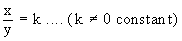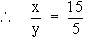Home MonkeyNotes Printable Notes Digital Library Study Guides Study Smart Parents Tips College Planning Test Prep Fun Zone Help / FAQ How to Cite New Title Request

 6.3 Variation It is the relation between a set of values of one variable and a set of values of other variables. Direct Variation: If variables x and y are such that for any corresponding values of these variables, we have the value of y/x as constant, we say that y varies directly as x or y is proportional to x or the variation in y is the same as the variation in x. In symbols, this is expressed as same as the variation in x. i.e. y µ x If the constant value of y/x is denoted by k then k is called constant of proportionality or constant of the variation. So, when y µ x, we have y = kx for some k and when for some k, y = kx, we have y µ x. Example If x µ y and x = 15 when y = 5 find : i) y when x = 9 ii) x when y = 8 Solution : x µ y . Therefore,When x = 15, y = 5= 3        = kNow i) Substitute x = 9 in x = 3y \ 9 = 3y \ y = 3 ii) Substitute y = 8 in x = 3y \ x = 3 ´ 8 = 24 Index 6.1 Relations 6.2 Functions 6.3 Variation Chapter 7
All Contents Copyright © All rights reserved.
Further Distribution Is Strictly Prohibited.

 Search: All Products Books Popular Music Classical Music Video DVD Toys & Games Electronics Software Tools & Hardware Outdoor Living Kitchen & Housewares Camera & Photo Cell Phones Keywords: